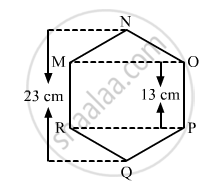Advertisement Remove all ads

# Find the Area of the Following Regular Hexagon. - Mathematics

Sum

Find the area of the following regular hexagon.Advertisement Remove all ads

#### Solution

The given figure is:
Join QN.It is given that the hexagon is regular. So, all its sides must be equal to 13 cm.
Also, AN = BQ
QB+BA+AN = QN
AN+13+AN = 23
2AN = 23-13 = 10
$AN =\frac{10}{2}= 5 cm$
Hence, AN = BQ = 5 cm
Now, in the right angle triangle MAN:
${MN}^2 {=AN}^2 {+AM}^2$
${13}^2 {=5}^2 {+AM}^2$
${AM}^2 =169-25=144$
$AM=\sqrt{144}=12cm.$
$\therefore OM = RP = 2\times AM = 2\times12 = 24 cm$
Hence, area of the regular hexagon = (area of triangle MON)+(area of rectangle MOPR)+(area of triangle RPQ)
$=(\frac{1}{2}\times OM\times AN)+(RP\times PO)+(\frac{1}{2}\times RP\times BQ)$
$=(\frac{1}{2}\times24\times5)+(24\times13)+(\frac{1}{2}\times24\times5)$
$=60+312+60$
${=432 cm}^2$
Is there an error in this question or solution?
Advertisement Remove all ads

#### APPEARS IN

RD Sharma Class 8 Maths
Chapter 20 Mensuration - I (Area of a Trapezium and a Polygon)
Exercise 20.3 | Q 5 | Page 29
Advertisement Remove all ads

#### Video TutorialsVIEW ALL 

Advertisement Remove all ads
Share
Notifications

View all notifications

Forgot password?
Course